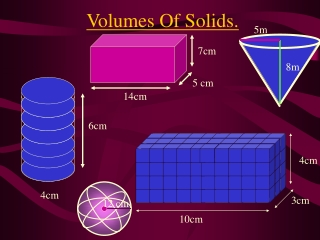Download PresentationVolumes Of Solids.# Volumes Of Solids. - PowerPoint PPT Presentation

Download Presentation##### Volumes Of Solids.

Download Policy: Content on the Website is provided to you AS IS for your information and personal use and may not be sold / licensed / shared on other websites without getting consent from its author. While downloading, if for some reason you are not able to download a presentation, the publisher may have deleted the file from their server.

- - - - - - - - - - - - - - - - - - - - - - - - - - - E N D - - - - - - - - - - - - - - - - - - - - - - - - - - -
##### Presentation Transcript

1. 5m 8m Volumes Of Solids. 7cm 5 cm 14cm 6cm 4cm 4cm 3cm 12 cm 10cm

2. What Is Volume ? The volume of a solid is the amount of space inside the solid. Consider the cylinder below: If we were to fill the cylinder with water the volume would be the amount of water the cylinder could hold:

3. 1cm 1cm 1cm Measuring Volume. Volume is measured in cubic units. Here is a cubic centimeter It is a cube which measures 1cm in all directions. We will now see how to calculate the volume of various shapes.

4. 4cm 3cm 10cm 10cm 3cm Volumes Of Prisms. Look at the rectangular prism below: We must first calculate the area of the base of the rectangular prism: The base is a rectangle measuring 10cm by 3cm:

5. 4cm 3cm 10cm 10cm 3cm Area of a rectangle = base x height Area = 10 x 3 Area = 30cm2 We now know we can place 30 centimeter squares on the base of the rectangular prism. But we can also place 30 cubic centimeters on the base to fill the prism:

6. 4cm 3cm 10cm We have now have to find how many layers of 1cm cubes we can place in the rectangular prism: We can fit in 4 layers. Volume = 30 x 4 Volume = 120cm3 That means that we can place 120 of our cubes measuring a centimeters in all directions inside our rectangular prism.

7. 4cm 3cm 10cm We have found that the volume of the rectangular prism is given by: Volume = 10 x 3 x 4 = 120cm3 This gives us our formula for the volume of a cuboid: Volume = Area of the Base X Height V=Bh for short.

8. 6cm 4cm Consider the cylinder below: The Volume Of A Cylinder. It has a height of 6cm . What is the size of the radius ? 2cm Volume = Area of base x height What shape is the base? Circle Calculate the area of the base: A =  r 2 A = 3.14 x 2 x 2 The formula for the volume of a cylinder is: V = Bh B = area of the base ( r 2) h = height. A = 12.56 cm2 Calculate the volume: V =  r 2 x h V = 12.56 x 6 V75.36 cm3

9. D D H H Volume Of A Cone. Consider the cylinder and cone shown below: The diameter (D) of the top of the cone and the cylinder are equal. The height (H) of the cone and the cylinder are equal. If you filled the cone with water and emptied it into the cylinder, how many times would you have to fill the cone to completely fill the cylinder to the top ? This shows that the cylinder has three times the volume of a cone with the same height and radius. 3 times.

10. r h B= area of the base h = height The experiment on the previous slide allows us to work out the formula for the volume of a cone: The formula for the volume of a cylinder is : V = Bh We have seen that the volume of a cylinder is three times more than that of a cone with the same diameter and height . The formula for the volume of a cone is:

11. (2) (1) 18m 13m 6m 9m Calculate the volume of the cones below:

12. Spheres Definition: In space, the set of all points that are a given distance from a given point, called the center. A sphere is formed by revolving a circle about its diameter.

13. r Volume of Sphere Volume (V) = 12 cm Example: Find the surface area and volume of the sphere. Volume= 2304π cm3  7238.23 cm3